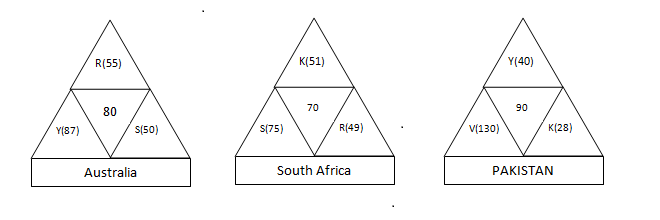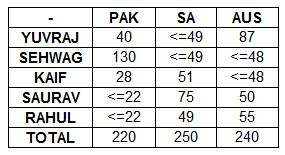### CAT 2004 Question Paper Question 59

Instructions

Directions for the following four questions: Answer the questions on the basis of the information given below.Coach John sat with the score cards of Indian players from the 3 games in a one-day cricket tournament where the same set of players played for India and all the major batsmen got out. John summarized the batting performance through three diagrams, one for each games. In each diagram, the three outer triangles communicate the number of runs scored by the three top scores from India, where K, R, S, V, and Y represent Kaif, Rahul, Saurav, Virender, and Yuvraj respectively. The middle triangle in each diagram denotes the percentage of total score that was scored by the top three Indian scorers in that game. No two players score the same number of runs in the same game. John also calculated two batting indices for each player based on his scores in the tournaments; the R-index of a batsman is the difference between his highest and lowest scores in the 3 games while the M-index is the middle number, if his scores are arranged in a non-increasing order.

Question 59

# Which of the players had the best M-index from the tournament?

SolutionSaurav has the best M-index .

### View Video Solution

• All Quant CAT Formulas and shortcuts PDF
• 30+ CAT previous papers with solutions PDF